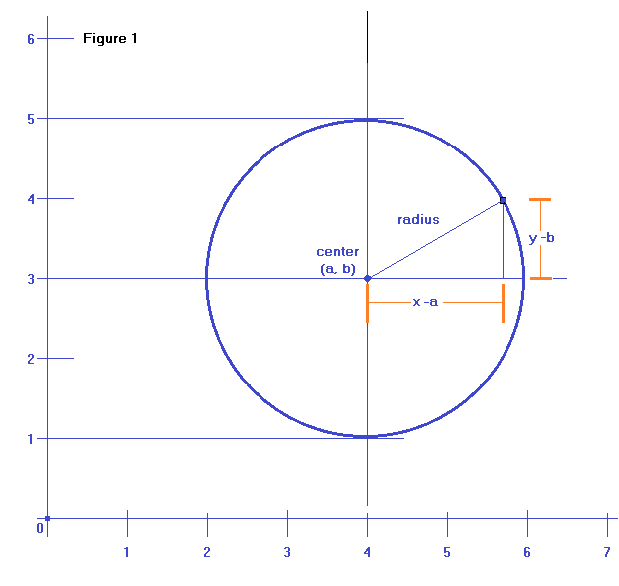Circle Equations

 A circle is a two-dimensional shape whose points are all equidistant from the center. All circles with centers located at the origin (0, 0) have the equation: x² + y² = r² Where r is the radius of the circle. So, a circle whose center is located at the origin (0, 0) with a radius of 4 has the equation: x² + y² = 16 Standard Form of a Circle's Equation A circle whose center is located at point (a, b) having a radius of length r has the equation: (x -a)² + (y -b)² = r² This is called the standard form of a circle's equation.       (This is sometimes called the center-radius form). A circle's center coordinates and radius can be easily determined from the standard form. A circle's center would be found at point   (a, b)   and its radius equals the   square root of r². So, for example, a circle with the equation     (x -2)² + (y -1)² = 25 Would have its center located at   (2, 1) and   would have a radius equal to the   square root of 25 or 5. A circle with the equation     (x +2)² + (y -1)² = 36 Would have its center located at   (-2, 1) and   would have a radius equal to the   square root of 36 or 6. General Form of a Circle's Equation Multiplying out a circle's standard form:   (x -a)² + (y -b)² = r² produces the general form of a circle's equation:  x² + y² + cx + dy + e = 0 Figure 1 below shows a circle whose center is at point (4, 3) with a radius of 2. How do we find the standard form equation of this circle? We put the center coordinates (4, 3) into the a and b variables and we square the radius for r² (x -4)² + (y -3)² = 4 By multiplying this out, we get the general form x² -8x + 16 +y² -6y + 9 = 4 x² +y² -8x -6y +21 = 0Going From General Form To Standard Form How do you convert a general form equation into a standard form equation? 1) Let's take this general form equation as an example. x² +y² +16x -18y -145 = 0 2) Set the equation up in this manner and move the "non-x" term to the right. (x  )² + (y  )² = 145 3) Looking at the equation in step (1), take the x coefficient (16)  and the y coefficient (-18)     divide both these numbers by two, giving us (8, -9)   Insert these numbers into equation 2, paying attention to the signs. (x + 8)² + (y - 9)² = 145 4) Finally, take the numbers from step 3 (8, -9), square them (64, 81) then add both these numbers to 145, giving us the finished equation:   (x + 8)² + (y - 9)² = 290 A calculator that computes a circle's equation after 3 points have been input is located here.